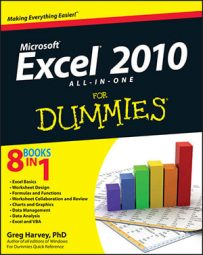##### Excel 2010 All-in-One For DummiesExcel 2010 offers an assortment of counting functions — including COUNT, COUNTA, COUNTBLANK, and COUNTIF — in the Statistical category that enable you to count the number of cells that contain numeric values, that are nonblank (and thus contain entries of any kind), or whose values meet the criteria that you specify. You can find these functions on the Statistical continuation menu accessed from the More Functions button's drop-down menu on the Formulas tab.

Excel's COUNTIF function lets you count cells in a range only when they meet a certain condition. The COUNTIF function takes two arguments and uses the following syntax:

`=COUNTIF(range,criteria)`

The range argument specifies the range of cells from which the conditional count is to be calculated. The criteria argument specifies the condition to use. You can express this argument as a number, expression, or text that indicates which cells to count. When specifying a number for the criteria argument, you don't have to enclose the number in quotes. For example, in a cell range named table_data, to count the number of entries that contain the number 5, you enter the following COUNTIF formula:

`=COUNTIF(table_data,5)`

However, when specifying an expression or text as the criteria argument, you must enclose the expression or text in closed quotes, as in "=5", ">20", or "New York". So, if you want to use COUNTIF to find out how many cells in the table_data range have values greater than 5, you enter this version of the COUNTIF function:

`=COUNTIF(table_data,">5")`

When you want to use the COUNTIF function to find out the number of cells whose contents are equal to the contents of a particular cell in the worksheet, you just add the cell reference as the function's criteria argument. For example, if you want to count the number of cells in the table_data range that are equal to the contents of cell B3 in the worksheet, you enter this formula:

`=COUNTIF(table_data,B3)`